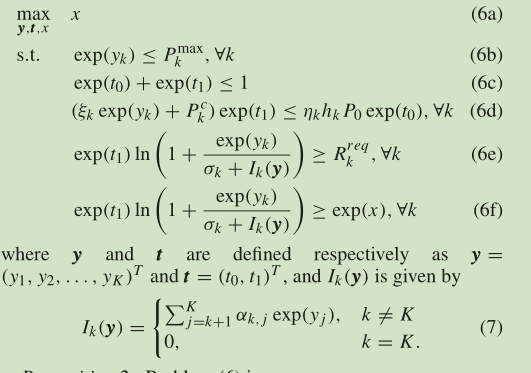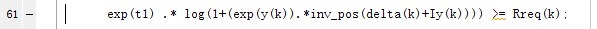# How to express exp(x).*log(1+exp(y).*inv_pos(z))?

hello,I’m trying to sovle this problem but something is wrong with the formula(6e):I try to express it like:Later I find that the formula like x*log(y) is not allowed in cvx,so I tryed this one:

and it doesn’t work ,either.

(the formula (6e) is convex)

Please show us your proof that (6e) is convex. I’m not saying it isn’t a convex constraint, but please show us that it is. When you do so, please consider carefully the guidance provided in Why isn’t CVX accepting my model? READ THIS FIRST! .

1 Like

okay,of course,the proof is writen below:

I am sorry that I just started learning this. I have tried to find it but still can’t solve this problem independently.

CVX has a log_sum_exp function

help log_sum_exp
log_sum_exp log(sum(exp(x))).
log_sum_exp(X) = LOG(SUM(EXP(X)).

``````When used in a CVX model, log_sum_exp(X) causes CVX's successive
approximation method to be invoked, producing results exact to within
the tolerance of the solver. This is in contrast to LOGSUMEXP_SDP,
which uses a single SDP-representable global approximation.

If X is a matrix, LOGSUMEXP_SDP(X) will perform its computations
along each column of X. If X is an N-D array, LOGSUMEXP_SDP(X)
will perform its computations along the first dimension of size
other than 1. LOGSUMEXP_SDP(X,DIM) will perform its computations
along dimension DIM.

Disciplined convex programming information:
log_sum_exp(X) is convex and nondecreasing in X; therefore, X
must be convex (or affine).

cvx/log_sum_exp
``````

So your difficulty will be dealing with the 2nd term of LHS. The proof provided for convexity of that term doesn’t immediately suggest to me a formulation which could be entered into CVX.

Perhaps one of the smarter forum readers will come along and suggest a formulation which couldl work, presuming that such a formulation exists (but you might have to wait a few days, since some of the cleverest readers don;t visit the forum every day)… Note that not all convex models can be entered into CVX, but an awful lot can, if the modeler is clever enough.

1 Like

Emmm,I just saw your reply in the usage of the function log_sum_exp when I visited the forum, so I tried to use this function before, but cvx still can’t pass this.
Thanks for your sharing and now I realize that not all convex models can be entered into CVX.
Sorry , I am just beginning to use cvx and I am really appreciate this forum and your discussions.
But the DCP rule sets are still confusing me.
I can’t find a good solution to make cvx pass it.

It would be better if you started trying to use CVX on some simpler, easier problems. After you gain experience, then you can try to solve some more difficult problems. You can try reading through lots of forum threads, some of which may help you understand better how to use and not use CVX effectively.

1 Like

Okay, I am realllly appreciate your suggestions, thank you very much for taking your time to help me.

Would you reveal author and title of that paper/book.

I am interested in if it can be posed using the exponential cone but would like to have he complete source.

1 Like

Convexity of Fairness-Aware Resource Allocation in Wireless Powered
Communication Networks
Chongtao Guo, Bin Liao, Lei Huang, Senior Member, IEEE, Qiang Li, and Xin Lin

After a quick read of the paper and a discussion with Michal Adamaszek, then we believe

• It cannot be formulated so CVX will accept it.
• It cannot be formulated as an exponential cone problem.

We do not have a proof of the two statements so we may be wrong. In fact we hope history will prove us wrong.

1 Like

The “proof” is that @Michal_Adamaszek is the wizard of conic reformulation…

Actually, that is not a scientific proof, but is a practical engineering “proof”, meaning it is highly unlikely a poster will come along any time soon and provide a formulation which would be accepted by CVX.

Perhaps the Andersen-Adamaszek Hypothesis will take its place in the annals of great unproved mathematical conjectures, alongside the Riemann Hypothesis and P = NP. . Indeed, the only hope to prove or disprove this conjecture may be if a @Michal_Adamaszek 2.0 is released.

The only other suggestion I can offer to YC2017W is to contact the authors of the paper and ask them if they know of any conic formulation or any way of reformulating which would be acceptable to CVX.

Maybe I should write an email to the original author, hope he can see and reply. Anyway,I’m really grateful to Mark_L_Stone and Erling, thank you very much for sharing your ideas with me .I would share the author’s idea if he would like to share his solutions .